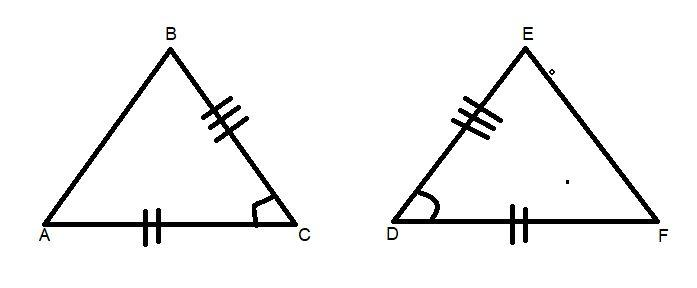Courses
Courses for Kids
Free study material
Free LIVE classes
MoreLIVE
Join Vedantu’s FREE Mastercalss

# Which condition of congruence proves that the following pair of triangles are congruent ?$A.$ A.A.S$B.$ S.S.S$C.$ S.A.S$D.$ A.S.AVerified
362.1k+ views
Hint: Here we go through by writing the conditions that are given through the diagram and after writing them we get to know that by which condition of congruence the triangles are congruent.

Here the given triangle is $\vartriangle ABC$ and $\vartriangle DEF$.
In $\vartriangle ABC$ and $\vartriangle DEF$.
Angle $\angle ACB = \angle FDE$ (Given in the figure).
Therefore $\vartriangle ABC \cong \vartriangle DEF$ According to S.A.S condition of congruence.